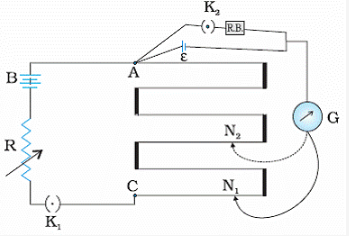# Write the principle of working of a potentiometer. Describe briefly

Write the principle of working of a potentiometer. Describe briefly, with the help of a circuit diagram, how a potentiometer is used to determine the internal resistance of a given cell.

Principle of working: The voltage drop along the wire is directly proportional to the length of the wire. The potentiometer works without drawing any current from the voltage source.We can also use a potentiometer to measure internal resistance of a cell. For this the cell (emf E) whose internal resistance ( r ) is to be determined is connected across a resistance box through a
Key K2, as shown in the figure.

With key K2 open, balance is obtained at length L1 (AN1).

Then,
E = ΦL1

When key K2 is closed, the cell sends a current (I ) through the resistance box ®. If V is the terminal potential difference of the cell and balance is obtained at length L2 (AN2), then
V = ΦL2
So, we have E/V = L1/L2
But, E= I (r + R) and V = IR. This gives
E/V = (r + R)/R

On comparing the above equations, we get,
(R + r)/R = L1/L2Using the above equation, we can find the internal resistance of a given cell.